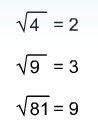## Sunday, February 1, 2009

### Mathematics - Indices and Algebra (Part 4) - SurdsExpressions with Indices, surds, exponentials and powers are the inverse of logarithm.
Indices and surds has real and known number.
Exponentials has unknowns, for example "X".
Logarithm of a number means the "exponential" of the base.
The flying pair of cranes is from http://www.cpedu.org/bbs/attachments/dvbbs/2006-6/200662918393174770.jpg

Normally in indices we come across square root of numbers giving results which are evaluated as exact number, just like the following.However there are also square root of numbers that do not evaluate exactly. The examples below shows some of those irrational results also called irrational roots.Numerical expressions which contain irrational roots are called surds.
Examples of surds. Conjugate surds exist too. They are a similar pair of expressions but one is with a negative and the other is with a positive irrational part.Reference